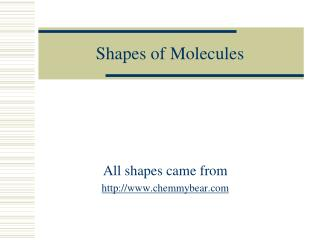Download PresentationShapes of Molecules

# Shapes of Molecules

Download Presentation## Shapes of Molecules

- - - - - - - - - - - - - - - - - - - - - - - - - - - E N D - - - - - - - - - - - - - - - - - - - - - - - - - - -
##### Presentation Transcript

1. Shapes of Molecules All shapes came from http://www.chemmybear.com

2. VSEPR theory • Valence shell electron pair repulsion theory • Simply stated it says- all atoms and electrons pairs will stay as far apart from one another as possible in 3-D space. • This is because electrons and the electron shells of each atom are negative and therefore repel each other. • We will only deal with the simple shapes around onecenter atom

3. So you have to imagine the shape we drew was in 3-D So something like methane - CH4 H H -C- H H Really looks like this This shape is called Tetrahedral The bond angle is 109.5o

4. Now if you have an unbonded pair of electrons… Something like ammonia NH3 The electron pair still repels the atoms so it looks like… .. H – N – H H This shape is called Trigonal Pyramidal The bond angle is 109.5o A tetrahedron without the top piece, or a triangle that is bent downwards

5. If you have 2 pairs of unbonded electrons Something like water H2O You get a shape that looks like .. :O – H H This shape is called Bent The bond angle is 109.5o A “V” shape

6. If you have 3 unbonded electron pairs Something like Chlorine gas, Cl2 .. .. :Cl-Cl: It looks exactly like we draw it .. .. This shape is called Linear A straight line

7. Steric number • Steric number- how many spots an atom has for pairs of electrons or bonds • steric number = bonds + unshared electron pairs • Every atom so far had a steric number of 4 • Steric number can go from 2-6 • Double/Triple bonds count as 1 bond. • *only get the steric number for the ONE center atom

8. Steric number of 3 Like BF3 or CH2O Will look like this F B-F F H C=O H This is called Trigonal Planar The bond angle is 120o A flat triangle

9. Steric number of 2 Like CO2 carbon dioxide O = C = O Is again a linear shape it looks exactly as it is drawn The bond angle is 180o

10. Steric number of 5 Like PF5 Phosphorus pentafluoride F F F P F F Would look like This is called Trigonal Bipyramidal

11. Steric # 5 with unbonded pairs of electrons • With one pair of unbonded electrons it is a See-saw shape (SF4, IOF4) • With two pairs of unbonded electrons it is T-Shaped (ClF3, BrF3) • With 3 unbonded pairs of electrons it is linear (XeF2)

12. Steric number of 6 Like SF6 sulfur hexafluoride Would look like F F F S F F F *really electronegative elements can break the octet rule This shape is Octahedral The bond angle is 90o

13. Steric # of 6 with unbonded pairs of electrons • With one unbonded pair of electrons it is square pyramidal. (XeOF4) • With two unbonded pairs of electrons it is square planar. (XeF4)

14. Table of all shapes

15. Effects of shape on polarity • Water is polar because it is bent CO2 is nonpolar because it is linear - O O C O - H H - + To be polar you need a positive end and a negative end + Negative at both ends cancel out